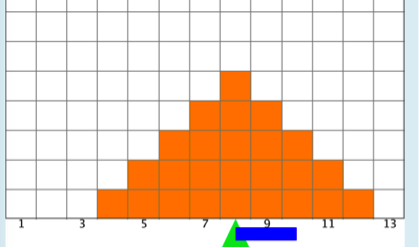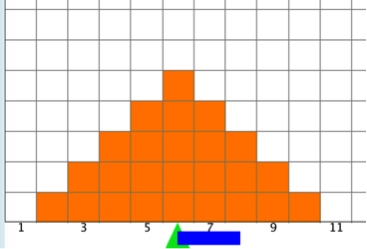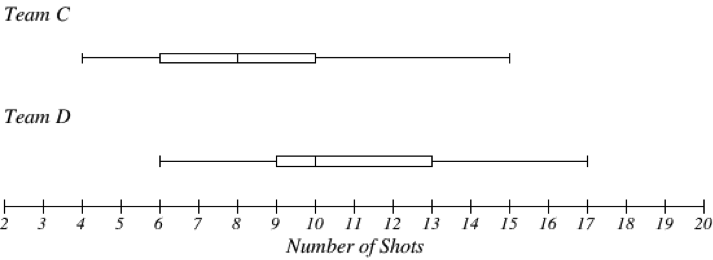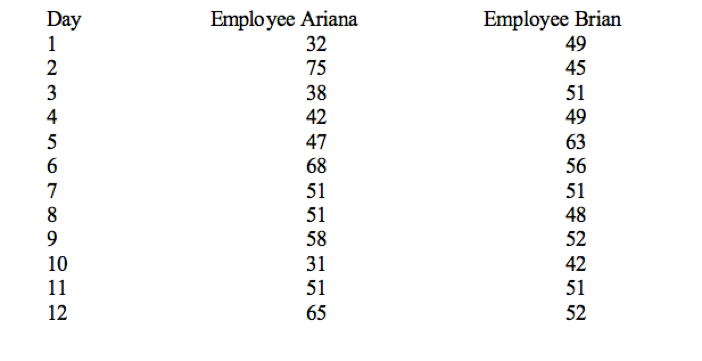# Descriptive Statistics

## Objective

Interpret the standard deviation and interquartile range.

## Common Core Standards

### Core Standards

?

• HSS-ID.A.2 — Use statistics appropriate to the shape of the data distribution to compare center (median, mean) and spread (interquartile range, standard deviation) of two or more different data sets.

• HSS-ID.A.4 — Use the mean and standard deviation of a data set to fit it to a normal distribution and to estimate population percentages. Recognize that there are data sets for which such a procedure is not appropriate. Use calculators, spreadsheets, and tables to estimate areas under the normal curve.

?

• 7.SP.B.3

• 7.SP.B.4

## Criteria for Success

?

1. Apply the standard deviation to contextual situations to determine consistency.
2. Apply the interquartile range to contextual situations to determine consistency.
3. Compare two data sets by analyzing the center and spread of the data sets.
4. Make conjectures about the number of standard deviations "allowed" in a data set. Describe why this makes sense.

## Tips for Teachers

?

This GeoGebra tool specifically shows the number of standard deviations and how moving points on the graph alters the mean, standard deviation, and median.

## Anchor Problems

?

### Problem 1

Below are the results that show the number of hours 50 babies slept in one night.How many babies have sleep habits within “one standard deviation” from the mean?

#### References

Standard Deviation Visually Represented in a Dotplot by Forest Fisher is made available by GeoGebra under the CC BY-NC-SA 3.0 license. Copyright © International GeoGebra Institute, 2013. Accessed June 21, 2017, 10:18 a.m..

### Problem 2

Two teams played 25 games of “cornhole” and charted the number of shots each team got in during a game.

Team A:                                               Team B:Mean: 8.0                                                       Mean: 6.0
Standard Deviation: 2.0                              Standard Deviation: 2.0

Which team is more consistent?

### Problem 3

After Team A and Team B were done playing their games, Team C and Team D took over. They altered the rules a bit for higher scoring capability.#### References

IMathAS Boxplot Grapher

Boxplot Grapher is made available by IMathAS under the GNU General Public License. Accessed June 26, 2017, 2:39 p.m..

## Problem Set

?

The following resources include problems and activities aligned to the objective of the lesson that can be used to create your own problem set.

• Use the GeoGebra applet to create other symmetrical distributions to illustrate the number of standard deviations needed to encompass the entire data set.
• Here is a sample problem:

The manager at a factory that makes pants is going to award one of the employees Employee of the Month. Below are the number of pants that each of two of the most productive workers have made over a period of 12 days.1. What are the measures of center for each of these employees? Describe in context of the problem.
2. Calculate an appropriate measure of spread for each employee. Describe the spread in context of the problem and name WHY you chose this measure of spread.
3. Who is the most consistent employee? How do you know? (Use mathematical vocabulary to describe “consistent.”)
4. Who do you think should receive the Employee of the Month award? Why?

?

Below are dot plots for three different data sets. The standard deviations for these three data sets are given in the following table. Looking at the dot plots and without calculating the standard deviations, match the data sets to the appropriate standard deviation.

Standard deviation A: 5.9
Standard deviation B: 3.3
Standard deviation C: 2.3Describe how the standard deviations affect consistency of a data set.